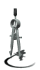Areas or Surfaces
 1 Acre = 43,560 square feet 4,840 square yards 0.405 hectare1 Are = 119.599 square yards 0.025 acre

 1 Hectare = 2.471 acres

 1 Square centimeter = 0.155 square inch

 1 Square decimeter = 15.5 square inches

 1 Square foot (feet) = 929.030 square centimeters 0.0929 square meters

 1 Square inch = 6.4516 square centimeters

 1 Square kilometer = 0.386 square mile 247.105 acres

 1 Square meter = 1.196 square yards 10.7638 square feet

 1 Square mile = 258.999 hectares

 1 Square millimeter = 0.002 square inch

 1 Square rod, square pole or square perch = 25.293 square meters

 1 Square yard = 0.836 square meters